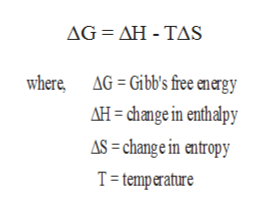# For the reactionCH4(g) + 2O2(g)CO2(g) + 2H2O(g)H° = -802.3 kJ and S° = -5.2 J/KThe maximum amount of work that could be done when 1.89 moles of CH4(g) react at 259 K, 1 atm is ________ kJ.

Question
45 views

For the reaction

CH4(g) + 2O2(g)CO2(g) + 2H2O(g)

H° = -802.3 kJ and S° = -5.2 J/K

The maximum amount of work that could be done when 1.89 moles of CH4(g) react at 259 K, 1 atm is ________ kJ.

check_circle

Step 1

Given : Change in enthalpy is -802.3 kJ, change in entropy is -5.2 J/K, temperature is 259 K and pressure is 1 atm.

Step 2

Gibbs free energy is defined as the maximum amount of work done by a system at a constant temperature and pressure.

Therefore, it means that Gibb\\'s free energy will always be equal to the total amount of work done.

Gibb\\'s free energy is related to change in enthaly and change in entropy as follows.help_outlineImage TranscriptioncloseAG ΔΗ- TAS AG= Gibb's free energy where AH change in enthalpy AS= change in entropy T temperature fullscreen
Step 3

Now, we put the given values in ...

### Want to see the full answer?

See Solution

#### Want to see this answer and more?

Solutions are written by subject experts who are available 24/7. Questions are typically answered within 1 hour.*

See Solution
*Response times may vary by subject and question.
Tagged in

### Chemistry